# Circular Dichroism Part I Introduction Circular Dichroism Circular

• Slides: 30Circular Dichroism Part I. Introduction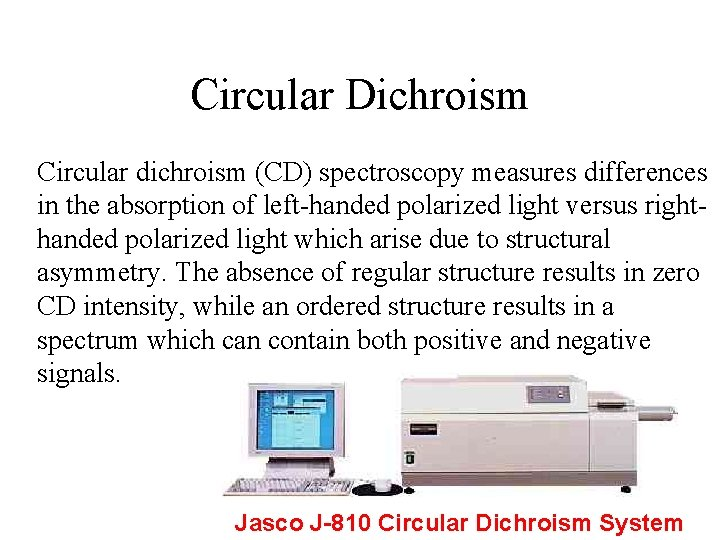Circular Dichroism Circular dichroism (CD) spectroscopy measures differences in the absorption of left-handed polarized light versus righthanded polarized light which arise due to structural asymmetry. The absence of regular structure results in zero CD intensity, while an ordered structure results in a spectrum which can contain both positive and negative signals. Jasco J-810 Circular Dichroism System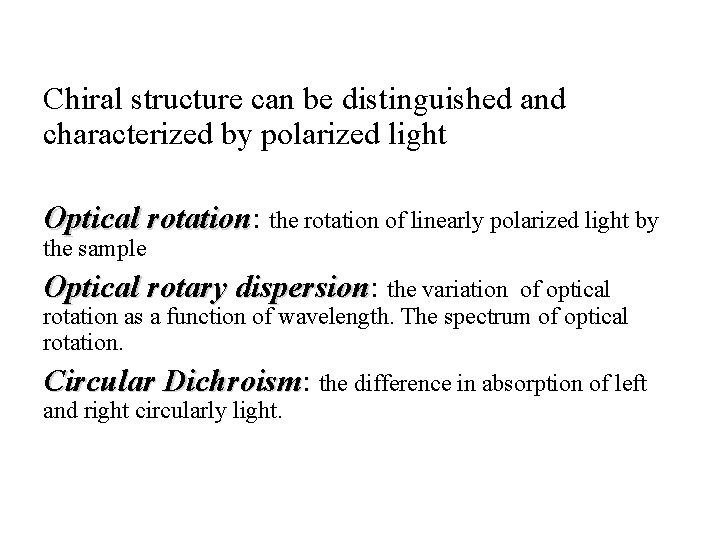Chiral structure can be distinguished and characterized by polarized light Optical rotation: rotation the rotation of linearly polarized light by the sample Optical rotary dispersion: dispersion the variation of optical rotation as a function of wavelength. The spectrum of optical rotation. Circular Dichroism: Dichroism the difference in absorption of left and right circularly light.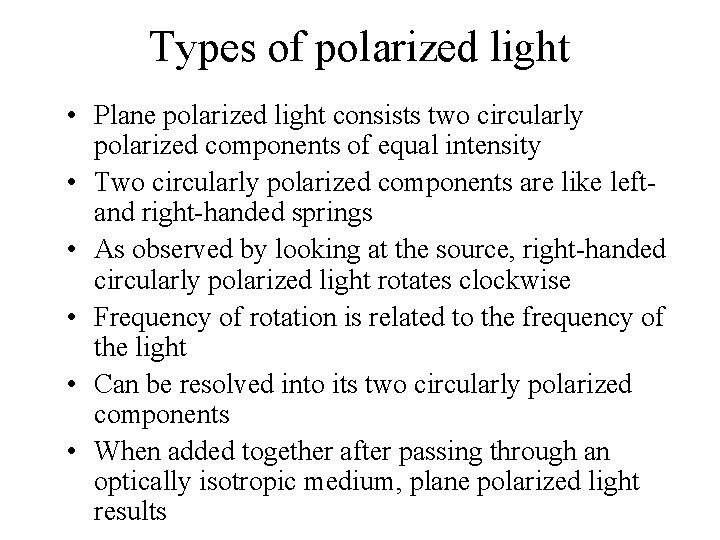Types of polarized light • Plane polarized light consists two circularly polarized components of equal intensity • Two circularly polarized components are like leftand right-handed springs • As observed by looking at the source, right-handed circularly polarized light rotates clockwise • Frequency of rotation is related to the frequency of the light • Can be resolved into its two circularly polarized components • When added together after passing through an optically isotropic medium, plane polarized light results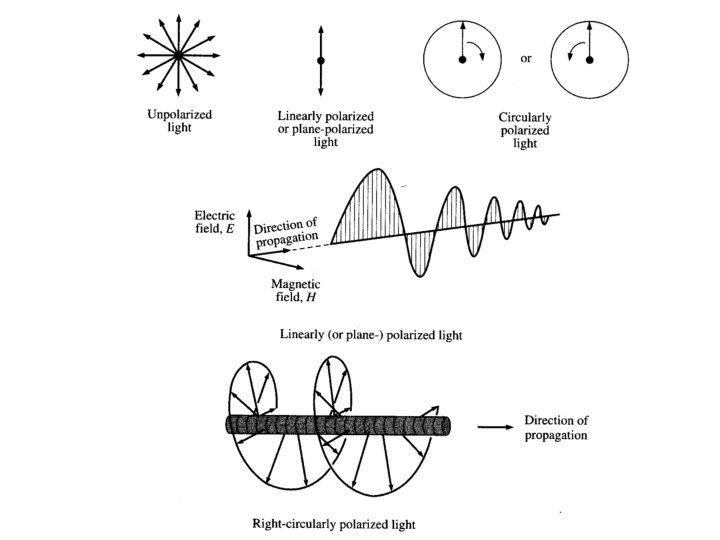Polarized Light Linear Polarized Light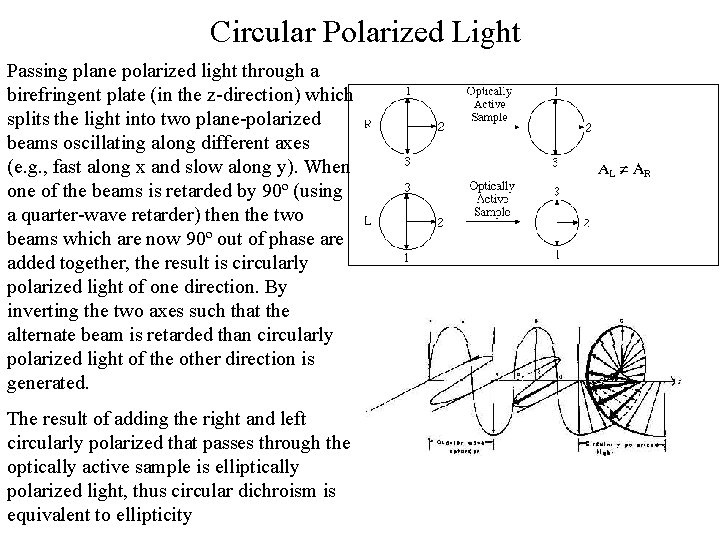Circular Polarized Light Passing plane polarized light through a birefringent plate (in the z-direction) which splits the light into two plane-polarized beams oscillating along different axes (e. g. , fast along x and slow along y). When one of the beams is retarded by 90º (using a quarter-wave retarder) then the two beams which are now 90º out of phase are added together, the result is circularly polarized light of one direction. By inverting the two axes such that the alternate beam is retarded than circularly polarized light of the other direction is generated. The result of adding the right and left circularly polarized that passes through the optically active sample is elliptically polarized light, thus circular dichroism is equivalent to ellipticityPolarized Light Circularly Polarized Light Left-handed right-handed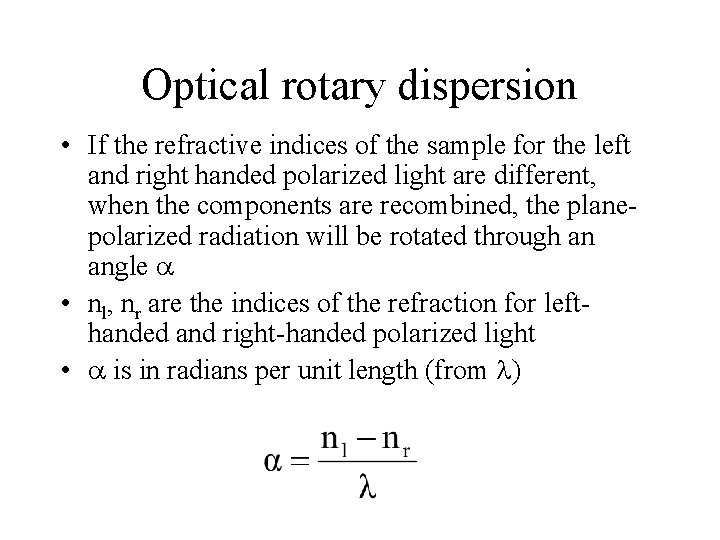Optical rotary dispersion • If the refractive indices of the sample for the left and right handed polarized light are different, when the components are recombined, the planepolarized radiation will be rotated through an angle • nl, nr are the indices of the refraction for lefthanded and right-handed polarized light • is in radians per unit length (from )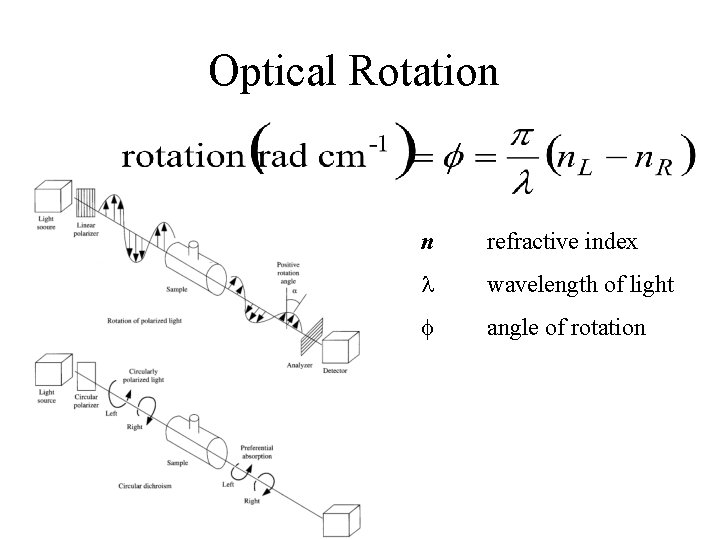Optical Rotation n refractive index wavelength of light f angle of rotation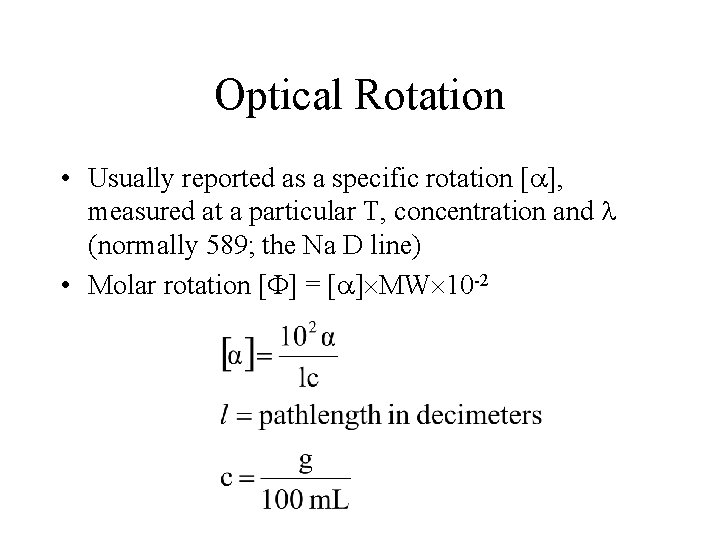Optical Rotation • Usually reported as a specific rotation [ ], measured at a particular T, concentration and (normally 589; the Na D line) • Molar rotation [ ] = [ ] MW 10 -2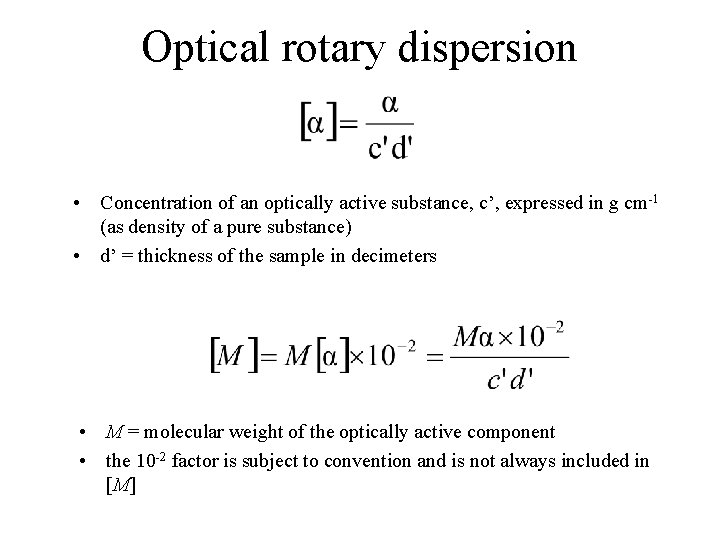Optical rotary dispersion • Concentration of an optically active substance, c’, expressed in g cm-1 (as density of a pure substance) • d’ = thickness of the sample in decimeters • M = molecular weight of the optically active component • the 10 -2 factor is subject to convention and is not always included in [M]Optical rotary dispersion • M = molecular weight of the optically active component • n. b. the 10 -2 factor is subject to convention and is not always included in [M]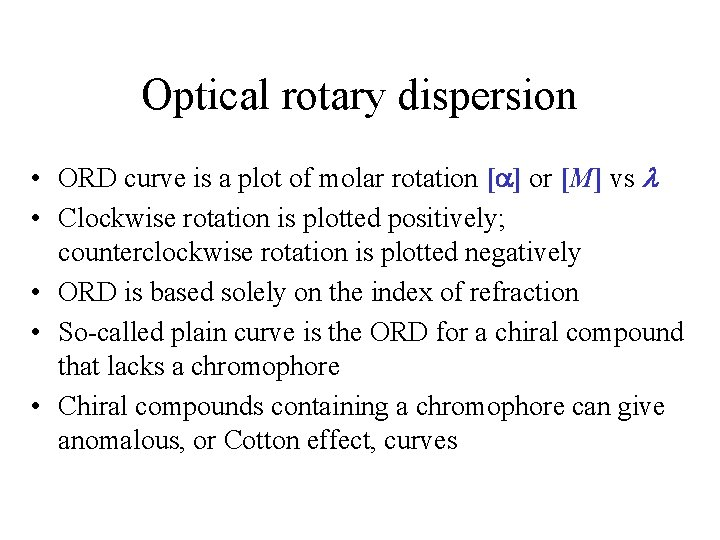Optical rotary dispersion • ORD curve is a plot of molar rotation [ ] or [M] vs • Clockwise rotation is plotted positively; counterclockwise rotation is plotted negatively • ORD is based solely on the index of refraction • So-called plain curve is the ORD for a chiral compound that lacks a chromophore • Chiral compounds containing a chromophore can give anomalous, or Cotton effect, curves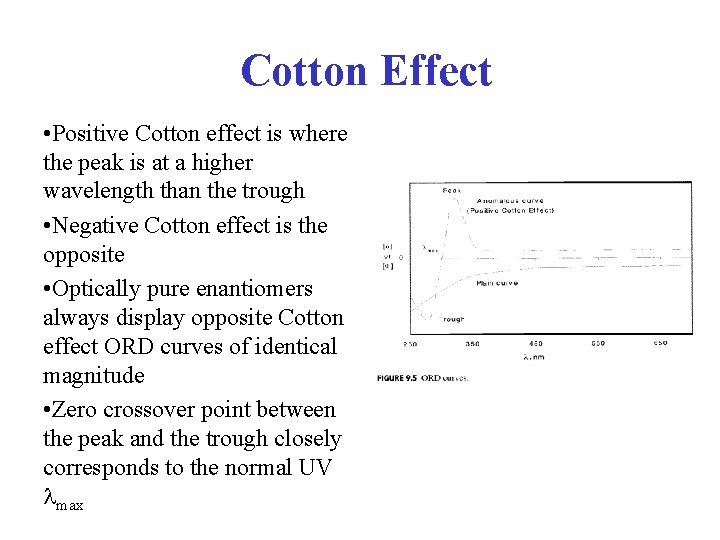Cotton Effect • Positive Cotton effect is where the peak is at a higher wavelength than the trough • Negative Cotton effect is the opposite • Optically pure enantiomers always display opposite Cotton effect ORD curves of identical magnitude • Zero crossover point between the peak and the trough closely corresponds to the normal UV maxCircular Polarized Light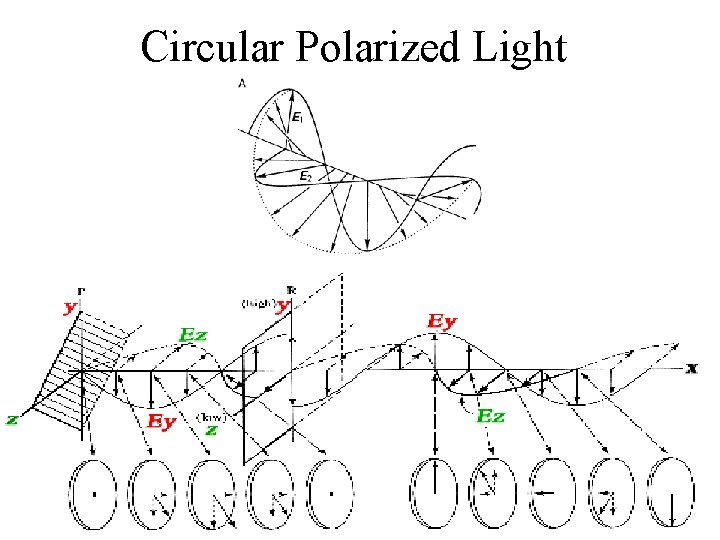Circular Polarized LightCircular dichroism • Measurement of how an optically active compound absorbs right- and left-handed circularly polarized light • All optically active compounds ex-hibit CD in the region of the appropriate absorption band • CD is plotted as l- r vs • For CD, the resulting transmitted radiation is not planepolarized but elliptically polarized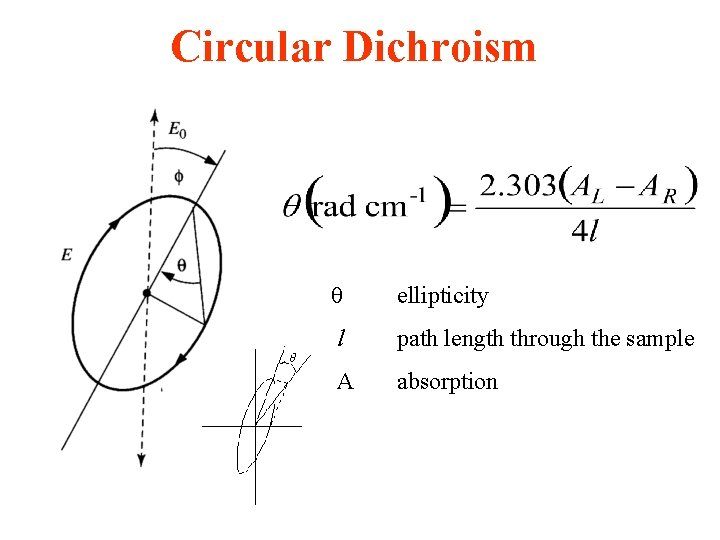Circular Dichroism ellipticity l path length through the sample A absorption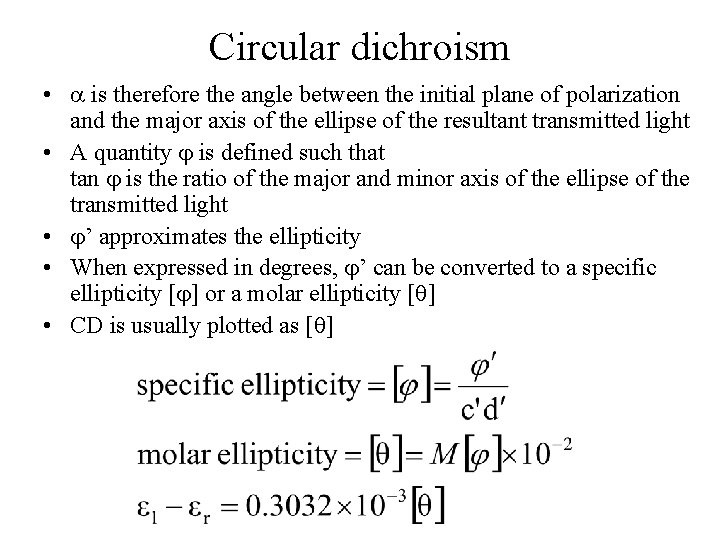Circular dichroism • is therefore the angle between the initial plane of polarization and the major axis of the ellipse of the resultant transmitted light • A quantity is defined such that tan is the ratio of the major and minor axis of the ellipse of the transmitted light • ’ approximates the ellipticity • When expressed in degrees, ’ can be converted to a specific ellipticity [ ] or a molar ellipticity [ ] • CD is usually plotted as [ ]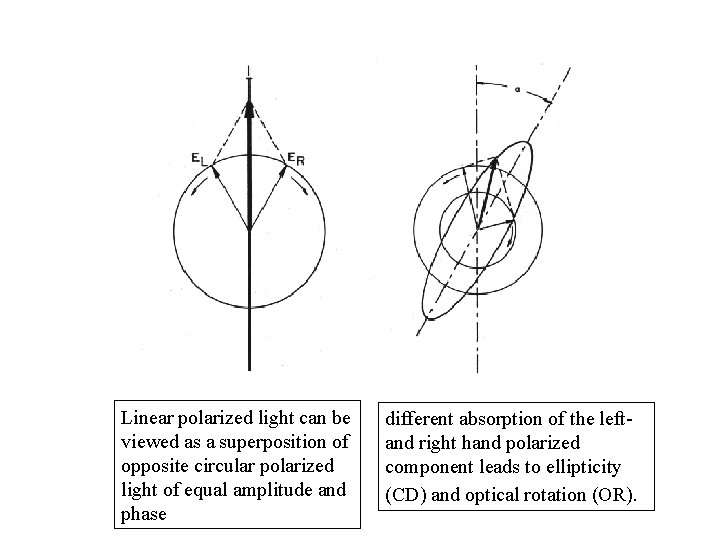Linear polarized light can be viewed as a superposition of opposite circular polarized light of equal amplitude and phase different absorption of the leftand right hand polarized component leads to ellipticity (CD) and optical rotation (OR).Circular Dichroism The difference between the absorption of left and right handed circularly-polarised light and is measured as a function of wavelength. CD is measured as a quantity called mean residue ellipticity, whose units are degrees-cm 2/dmol.ORD and CD • CD plots are Gaussian rather than S-shaped. • Positive or negative deflections depend on the sign of or [ ] and corresponds to the sign of the Cotton effect • ORD spectra are dispersive (called a Cotton effect for a single band) whereas circular dichroism spectra are absorptive. The two phenomena are related by the socalled König-Kramers transforms. • Maximum of the CD occurs at the absorption max • Where more than one overlapping Cotton effect, the CD may be easier to interpret than the ORD with overlapping S-shaped bands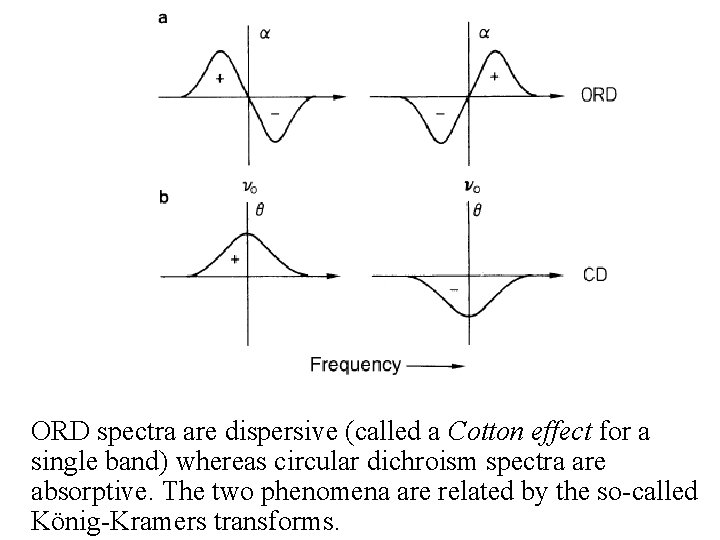ORD spectra are dispersive (called a Cotton effect for a single band) whereas circular dichroism spectra are absorptive. The two phenomena are related by the so-called König-Kramers transforms.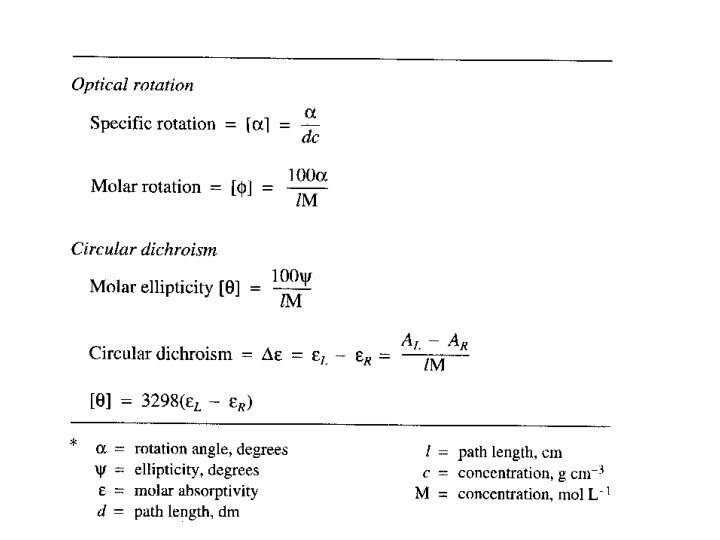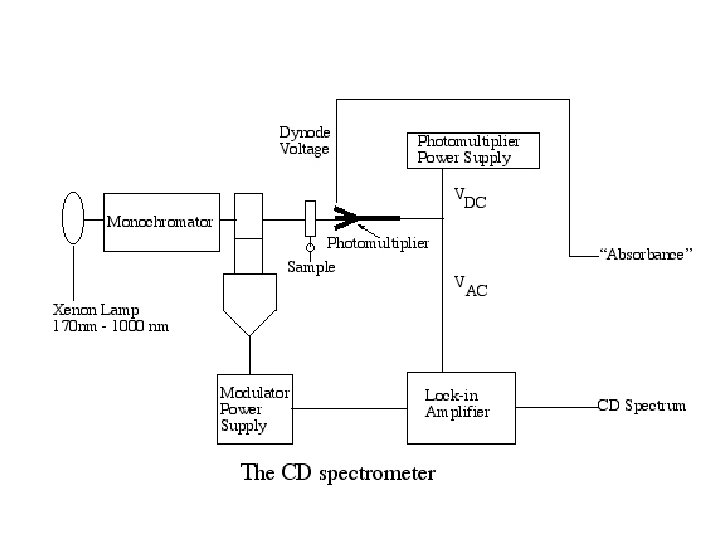Sample Preparation • Additives, buffers and stabilizing compounds: Any compound which absorbs in the region of interest (250 - 190 nm) should be avoided. • A buffer or detergent or other chemical should not be used unless it can be shown that the compound in question will not mask the protein signal.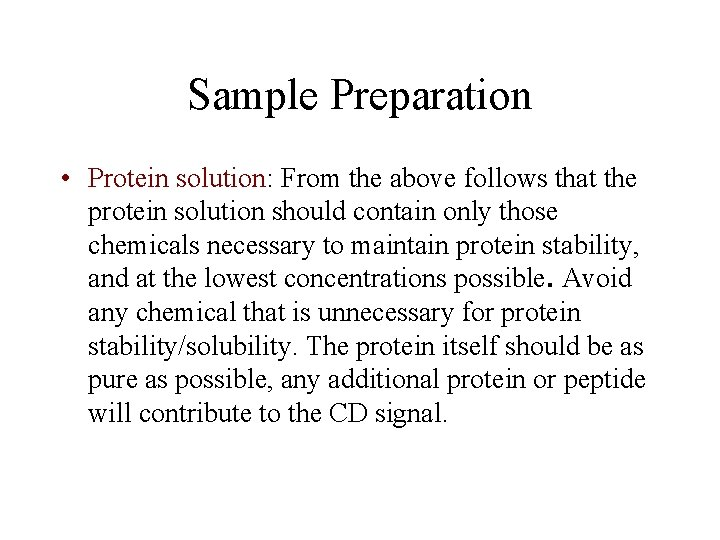Sample Preparation • Protein solution: From the above follows that the protein solution should contain only those chemicals necessary to maintain protein stability, and at the lowest concentrations possible. Avoid any chemical that is unnecessary for protein stability/solubility. The protein itself should be as pure as possible, any additional protein or peptide will contribute to the CD signal.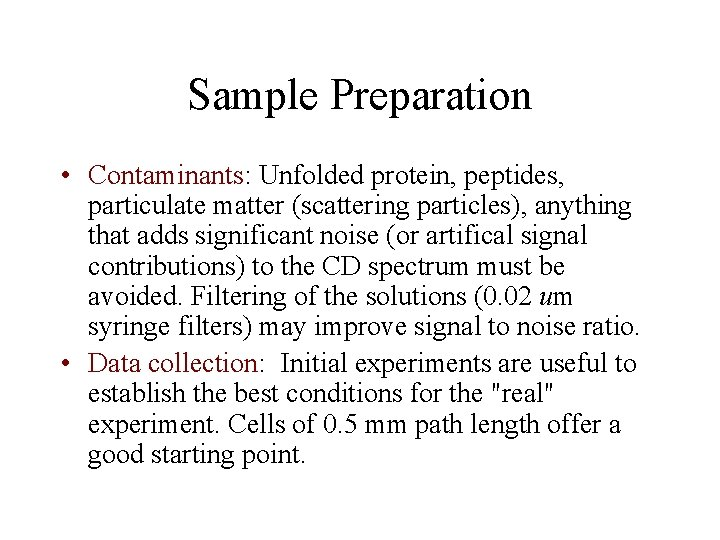Sample Preparation • Contaminants: Unfolded protein, peptides, particulate matter (scattering particles), anything that adds significant noise (or artifical signal contributions) to the CD spectrum must be avoided. Filtering of the solutions (0. 02 um syringe filters) may improve signal to noise ratio. • Data collection: Initial experiments are useful to establish the best conditions for the "real" experiment. Cells of 0. 5 mm path length offer a good starting point.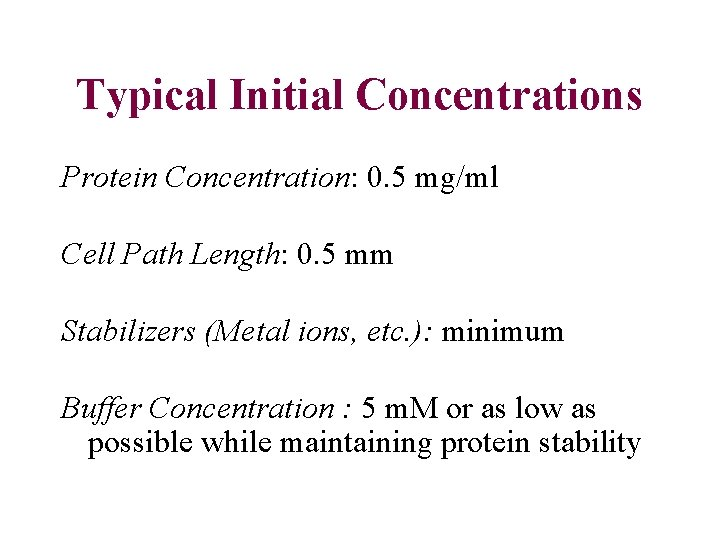Typical Initial Concentrations Protein Concentration: 0. 5 mg/ml Cell Path Length: 0. 5 mm Stabilizers (Metal ions, etc. ): minimum Buffer Concentration : 5 m. M or as low as possible while maintaining protein stability